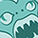## Simulate timeseries data with a SAS DATA Step and SAS Functions

Started ‎04-15-2020 by
Modified ‎04-15-2020 by
Views 4,468

In many cases you want to create simulated data for demonstration purposes or to verify features of certain methods, or you need simulated data to validate your SAS programs. In order to fit for the respective task, this data should not only be "just random data," but it should contain pattern and features that are needed for your task.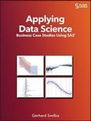This articles shows you can use a SAS DATA step with random number generators and a SAS Informat to simulate monthly time series data with specific patterns like trends, seasonal variation, breakpoints and outliers. It outlines options to analyze the course of the time series with analytical methods to identify breakpoints and outliers.

This tip is taken from the book Applying Data Science - Business Case Studies Using SAS

## Prework: Preparing a Lookup Table for the Seasonal Variation

If you want to introduce a specific monthly variation into your data, you could for example use a sequence of IF/THEN/ELSE or SELECT/WHEN statements. A more elegant and flexible solution is to prepare a SAS Informat with the monthly average values.

``````proc format;
invalue fl_mon
1 =438
2 =426
3 =516
4 =494
5 =506
6 =536
7 =566
8 =573
9 =478
10 =508
11 =479
12 =490;
run;``````

This INFORMAT is used with the INPUT function in the datastep to retrieve the respective value per month.

## Simulating the Data with a SAS DATA Step

The DATA step that creates the data is explained here step by step.

### Generating Data with a DO Loop

The following statements are used to create the data set FLIGHTS_SIMUL by using a DO loop to loop over the years from 1981 to 2000 and the months 1 to 12.

``````data flights_simul;
*** Initialize the seed for the random number generator;
call streaminit(20886); *** you can use any number;
format Date yymmp7. Passengers 8.;
drop year month;
do Year = 1981 to 2000; *** Loop over Years;
do month = 1 to 12;  *** Loop over Months;
*** Prepare the TIME Variable;
date = mdy(month,1,year);``````

Note that no SET statement is used, as no data set is used as input source. The data are created in the DATA step with a nested DO loop. The date variable is created with the MDY function from the month and the year value.

### Defining the Basic Form of the Time Series

In the next step, the seasonal variation, a linear trend, and a random variation is introduced into the data. Note that the scalar, 400, 40, and 1000 in the expressions are just arbitrary and are used to shift and re-scale the distribution of the values.

``````         *** Use the INPUT function to retrieve values from the INFORMAT;
passengers = (input(month, fl_mon.)-400)*40;``````

You see that the SAS informat FL_MON that was previously generated, is used to “query” the monthly averages.

• For this purpose the MONTH variable is used in the INPUT function with the informat as created above.
• The resulting value is the monthly average for the respective month.

A positive linear trend is introduced and random variation is added with the RAND function that generates a uniformly distributed number.

``````         *** Add a linear trend to the data;
passengers =  Passengers + (year-1981+1)*1000;
*** Add random variation to the data;
passengers = passengers + rand('uniform')*1000;``````

Note that the RAND function is used here as it should be the best practice to generate random numbers in SAS. This function uses the Mersenne-Twister algorithm and generates random numbers from sequences with a longer period. You could alternatively also use the RANUNI function.

### Adding Structural Changes and Outliers

The following statements are used to add structural changes and outliers in the data. A shift of +20% is introduced for the years 1986 and 1987.

``````         *** Add outliers and level shifts;
if year in (1986,1987) then passengers = passengers * 1.2;``````

The value in 1992 are cumulatively decreased by 300 for each month. The expression "Year in (1992)" shows a coding option to avoid an IF-statement. You receive the same output when using the IF-statement. There are situations where you might want write your value assignment as a one-line expression.

``         passengers =  Passengers + (year in (1992)) * (-month*300);``

Positive and negative outliers are introduced for certain months.

``````         if date = '01APR1997'd then passengers = passengers * 1.25;
if date = '01SEP1998'd then passengers = passengers * 0.8;
if date = '01APR1990'd then passengers = passengers * 1.2;``````

### Output the records and close the DATA step

Finally, the records are output and the DATA step is closed.

``````       *** Output the record;
output;
end;
end;
run;``````

You see that the SAS DATA step is very powerful to simulate your time series data and to specify different types of pattern in the data. You can thus easily generate your data for software demonstrations or test data for your analyses.

## Printing Selected Records

The following code prints the records for year 1992. This is the year where the monthly value was cumulatively decreased by 300 every month.

```proc print data=flights_simul;
where year(date) = 1992;
run;```

Output Window

``````                   Obs       Date    Passengers
133    1992.01        13962
134    1992.02        12558
135    1992.03        16658
136    1992.04        15133
137    1992.05        15567
138    1992.06        16605
139    1992.07        16903
140    1992.08        17028
141    1992.09        13077
142    1992.10        14298
143    1992.11        12073
144    1992.12        12421 ``````

## Plotting the Time Series

The following figure shows the plots of the time series. It was created with the following SAS statements.

``````proc sgplot data=flights_simul;
series x=date y=passengers;
run;``````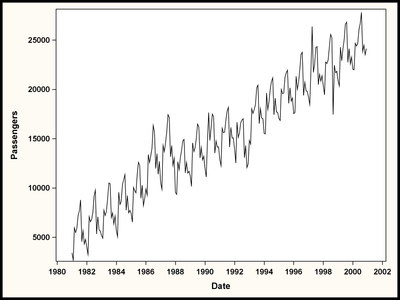## Running Further Analyses

This example is taken from case study 2 of my book, Applying Data Science - Business Case Studies Using SAS. In case study 2, you find an extensive discussion how to smooth time series data and to detect breakpoints and outliers with different SAS analytic procedures like PROC ADAPTIVEREG or PROC X13.

### Smoothing the Data with the EXPAND procedure

The data have been smoothed with a 12-month moving average using the CONVERT statement in the EXPAND procedure.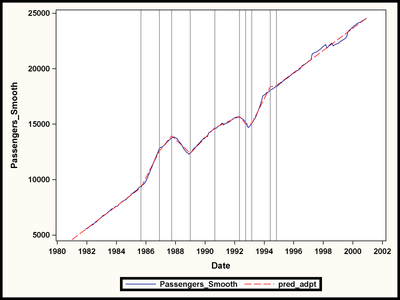### Detecting Breakpoints with the ADAPTIVEREG procedure

The ADAPTIVEREG procedure has been used to automatically identify the breakpoints in the data. You see that the method has been able to spot the inserted changes in the data.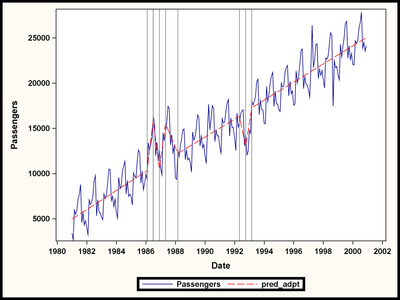### Detecting Outliers with the X13 procedure

The X13 procedure has been used to automatically identify the outliers in the data. You see that the method has been able to spot the inserted outliers in the data.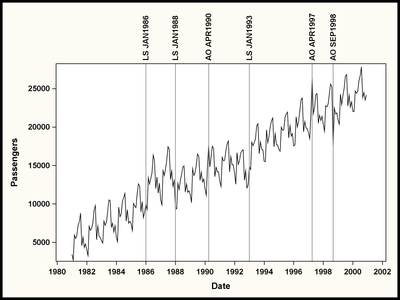Note that the reference lines have been automatically inserted into the graph based on the detected time points. A tip that explains this method is planned to be added to SAS Communities soon.

# Other articles in this series